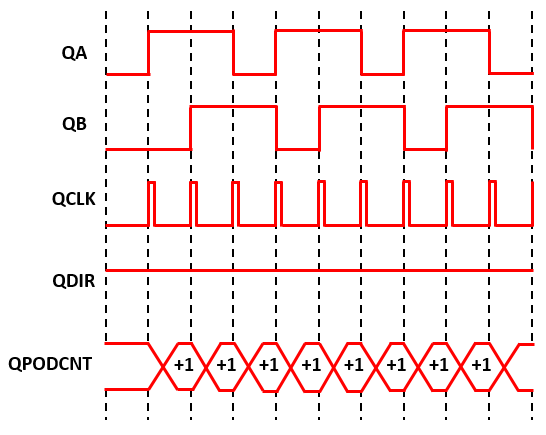Since R2020a

•Libraries:
Motor Control Blockset / Sensor Decoders
Motor Control Blockset HDL Support / Sensor Decoders

## Description

To calculate the angular position of the quadrature encoder (and the rotor) in either degrees, radians, or per-unit, the block uses one of the following methods.

• Difference between current encoder counter value and encoder counter value at the previous index pulse (when index pulse is available).

• Current encoder counter value (when index pulse is not available).

This figure shows a quadrature encoder disk with two channels (QEPA and QEPB) and an index pulse (QEPI):In this example, the timer driven by the QEP increments by four for each slit:### Equations

The block computes the angular position (in counts) of the quadrature encoder using the `Cnt` and `Idx` block inputs:

When Cnt ≥ Idx:

When Cnt < Idx:

When `Cnt` (an unsigned integer) exceeds the maximum value of the selected counter size, the block adds the necessary compensation internally.

When you clear the External index count checkbox, only `Cnt` input is available, therefore:

where:

• is the angular position of the quadrature encoder in counts.

• is the number of counts in one rotation cycle of the quadrature encoder (Counts per revolution = Encoder slits ⨯ Encoder counts per slit).

The block computes the output θm as:

Where, MaxPosition = 360 (degrees) or 2π (radians) or 1 (per-unit), based on the selected value of the Position unit parameter.

## Ports

### Input

expand all

Value that the quadrature encoder counter generates with respect to the slit-position. The port only accepts a scalar unsigned integer based on the Counter size parameter. For example, if you select `8 bits` for Counter size, the input data type must be uint8.

Data Types: `uint8` | `uint16` | `uint32`

Value that the quadrature encoder counter generated with respect to the slit-position at the time of the last index pulse. The port only accepts a scalar unsigned integer based on the Counter size parameter. For example, if you select `8 bits` for Counter size, the input data type must be uint8.

#### Dependencies

To enable this port, select the External index count parameter.

Data Types: `uint8` | `uint16` | `uint32`

Number of counts the quadrature encoder generates for each revolution.

CountsPerRev = Encoder slitsEncoder counts per slit

#### Dependencies

To enable this port, select the Use input port for computed parameters (run-time motor swap) parameter.

Data Types: `uint8` | `uint16` | `uint32` | `single` | `double` | `fixed point`

Specify the inverse of the number of counts the quadrature encoder generates for each revolution at this port. The Data type for 1/CountsPerRev input parameter defines the data type of this input.

#### Dependencies

To enable this port, select the Use input port for computed parameters (run-time motor swap) parameter.

Data Types: `single` | `double` | `fixed point`

Note

The data type of the input at the Cnt and Idx ports must be identical.

### Output

expand all

Angular position that the block computes based on the `Cnt` and `Idx` inputs.

Data Types: `single` | `double` | `fixed point`

## Parameters

expand all

The number of slits available in each phase of the quadrature encoder.

#### Dependencies

To enable this parameter, clear the Use input port for computed parameters (run-time motor swap) parameter.

The number of counts that the quadrature encoder generates for every slit. A count indicates a slit position. For example, select 4 (quadrature mode) if you want the encoder to generate four counts corresponding to 00, 10, 11, and 01 slit positions or values. If you select the quadrature mode:

Encoder counts per revolution (post-quadrature) = Encoder counts per slitEncoder slits

#### Dependencies

To enable this parameter, clear the Use input port for computed parameters (run-time motor swap) parameter.

Counter size signifies the register size of the counter used by the processor to count the quadrature encoder pulses. For example, when using TI’s C2000™ based hardware, it is the data type of the counter output of the eQEP block from C2000™ Microcontroller Blockset.

The block enables the Idx input port only if you select this parameter. The Idx input port expects a value equal to Cnt at the time of index pulse.

Unit of the angular position output.

The data type for the angular position output.

Note

The Quadrature Decoder block can display the warning message `'Wrap on overflow detected'` when needed.

The block enables the `CountsPerRev` and `1/CountsPerRev` input ports only if you select this parameter. When you select this parameter, the block also disables the Encoder slits and Encoder counts per slits parameters.

Specify the data type for the `1/CountsPerRev` input. If you specify the fixed-point data type, you can use this parameter to specify the precision of the `1/CountsPerRev` input.

#### Dependencies

To enable this parameter, select the Use input port for computed parameters (run-time motor swap) parameter.

## Version History

Introduced in R2020a